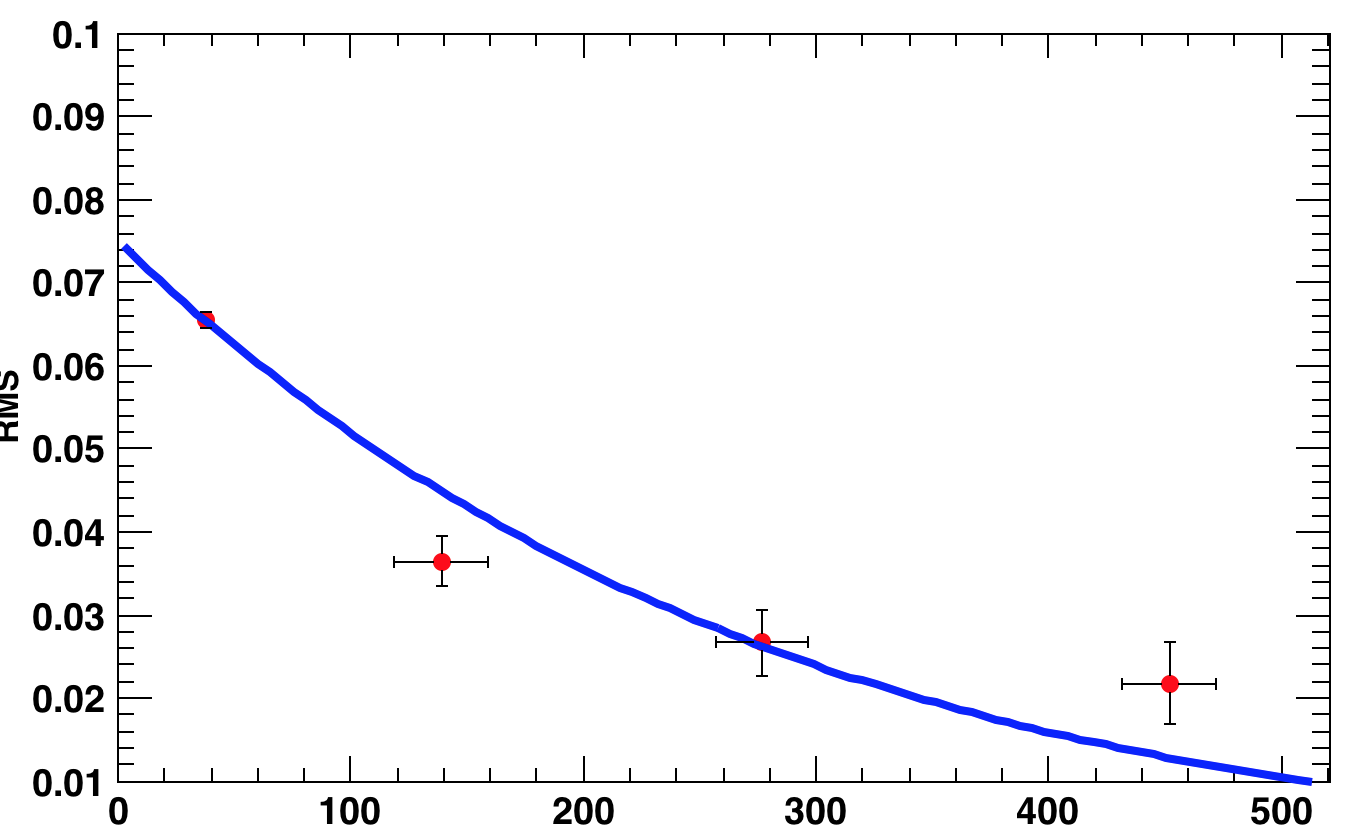# Fitting four points in terms of central limit theorem

Dear Experts,
I am new to the field of fitting the points. I need to check whether the given four points is the result of central limit theorem or not?

I am guessing that I should fit the point with gaussian function. But still I want to confirm it.

Hi @Surya001,

You seem to be wanting to test if the central limit theorem holds for your data. If it does, your data should indeed follow the Gaussian distribution. However, if you only have four points, fitting a Gaussian is complete overkill, because it has three parameters. What are your data, exactly?

1 Like

Hi @StephanH , Thank you for the response.

My 4 data points is actually four rms value taken from four different histograms. I am plotting rms values with respect to number of observations. I see that it(rms) is somewhat decreasing with the number of observations. Therefore, I was thinking to apply central limit theorem to check whether they fall into the fitting line.
(To test central limit theorem we need to fit with gaus right??)

I fitted with gaus and saw that they the points lies along the fitting line.I have uploaded the fit . Just want to know whether it is correct or not?

Thank you!!

I think that you should first check if the central limit theorem can possibly apply to your problem. It only applies if you add independent random variables from the same distribution (you can look for the definition of i.i.d., https://en.wikipedia.org/wiki/Independent_and_identically_distributed_random_variables
and also check the article on the CLT itself).
If you have four histograms, they need to be from same distribution, but independent measurements. Now the CLT applies.
In your case, since the mean value decreases, it probably doesn’t apply.

1 Like

Hmm … @StephanH

Thank you. Will have a look at it.

This topic was automatically closed 14 days after the last reply. New replies are no longer allowed.